## Pages

### Real Numbers and the Number Line

Natural Numbers – The set of counting numbers { 1, 2, 3, 4, 5, …}.
Whole Numbers – Natural numbers combined with zero  { 0, 1, 2, 3, 4, 5, …}.
Integers – Positive and negative whole numbers including zero {…,−5, −4, −3,−2, −1, 0, 1, 2, 3, 4, 5…}.
Rational Numbers – Any number of the form a/b where a and b are integers where b is not equal to zero.
Irrational Numbers – Numbers that cannot be written as a ratio of two integers.

When comparing real numbers, the larger number will always lie to the right of smaller numbers on a number line.  It is clear that 15 is greater than 5, but it might not be so clear to see that −5 is greater than −15.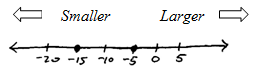Use inequalities to express order relationships between numbers.
<   "less than"
>   "greater than"
≤   "less than or equal to"
≥   "greater than or equal to"

It is easy to confuse the inequalities with larger negative values.  For example,
−120 < −10     “Negative 120 is less than negative 10.
Since −120 lies further left on the number line, that number is less than −10.  Similarly, zero is greater than any negative number because it lies further right on the number line.
0 > −59     "Zero is greater than negative 59."

Write the appropriate symbol, either < or >.List three integers satisfying the given statement. (Answers may vary.)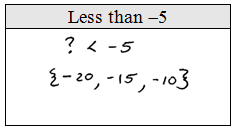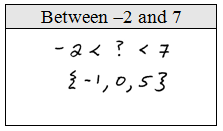Absolute Value – The distance between 0 and the real number a on the number line, denoted |a|. Because the absolute value is defined to be a distance, it will always be positive. It is worth noting that |0| = 0.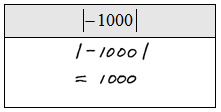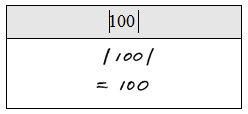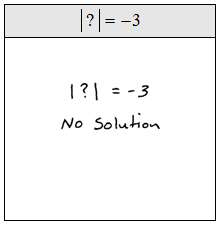Point of confusion: You may encounter negative absolute values like this −|3|. Notice that the negative is in front of the absolute value. Work the absolute value first, then consider the opposite of the result. For example,
−|3| = −3
−|−7| = −7

Believe it or not, the above are correct! Look out for this type of question on an exam.